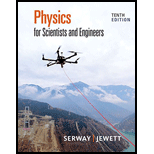Chapter 16, Problem 47APPhysics for Scientists and Enginee...

10th Edition
Raymond A. Serway + 1 other
ISBN: 9781337553278

Solutions

Chapter
SectionPhysics for Scientists and Enginee...

10th Edition
Raymond A. Serway + 1 other
ISBN: 9781337553278
Textbook Problem

A sinusoidal wave in a string is described by the wave function y = 0.150 sin   ( 0.800 x − 50.0 t ) where x and y are in meters and t is in seconds. The mass per length of the string is 12.0 g/m. (a) Find the maximum transverse acceleration of an element of this string. (b) Determine the maximum transverse force on a 1.00-cm segment of the string. (c) State how the force found in part (b) compares with the tension in the string.

(a)

To determine

The maximum transverse acceleration.

Explanation

The mass per length of the string is 12.0g/m.

The wave function of the string is given as,

y=0.150sin(0.800x50.0t)

The above expression is the standard form of the wave function,

y=Asin(kxwt).

Here, k is the wave constant, ω is the angular frequency and t is the time interval.

Formula to calculate thetransverse speed is,

v=dydt        (1)

Here, v is the transverse speed of the wave and dt is the time travel.

Substitute 0.150sin(0.800x50.0t) for y in equation (1) to get the v.

v=d(0.150sin(0.800x50.0t))dt=0.150cos(0.800x50.0t)(50)=7.5cos(0.800x50.0t)

For maximum transverse speed,

cos(0.800x50.0t)1

Therefore,the maximum transverse speed is,

v=7.5m/s

The negative sign is not considered for the transverse because transverse represents the negative direction

(b)

To determine

The maximum transverse force.

(c)

To determine

The comparison between maximum transverse force and tension in the string.

Still sussing out bartleby?

Check out a sample textbook solution.

See a sample solution

The Solution to Your Study Problems

Bartleby provides explanations to thousands of textbook problems written by our experts, many with advanced degrees!

Get Started

The freezing point of water is _________ K.

Introductory Chemistry: A Foundation

Match the concepts:

Human Biology (MindTap Course List)

What is the structure and function of a chloroplast?

Biology: The Dynamic Science (MindTap Course List)

Why cant marine organisms use the nitrogen gas dissolved in seawater?

Oceanography: An Invitation To Marine Science, Loose-leaf Versin